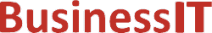O nás     Inzerce     KontaktSpolehlivé informace o IT již od roku 2011[Linux manuál]

# tgamma, tgammaf, tgammal: vypočítat funkci gama ()

Originální popis anglicky: tgamma, tgammaf, tgammal - compute gamma() function

Návod, kniha: POSIX Programmer's Manual

## STRUČNĚ

#include <math.h>

double tgamma(double x);

float tgammaf(float x);

long double tgammal(long double x);

## POPIS / INSTRUKCE

These functions shall compute the gamma() function of x.
An application wishing to check for error situations should set errno to zero and call feclearexcept(FE_ALL_EXCEPT) before calling these functions. On return, if errno is non-zero or fetestexcept(FE_INVALID | FE_DIVBYZERO | FE_OVERFLOW | FE_UNDERFLOW) is non-zero, an error has occurred.

## NÁVRATOVÁ HODNOTA

Upon successful completion, these functions shall return Gamma( x).
If x is a negative integer, a domain error shall occur, and either a NaN (if supported), or an implementation-defined value shall be returned.
If the correct value would cause overflow, a range error shall occur and tgamma(), tgammaf(), and tgammal() shall return ±HUGE_VAL, ±HUGE_VALF, or ±HUGE_VALL, respectively, with the same sign as the correct value of the function.
If x is NaN, a NaN shall be returned.
If x is +Inf, x shall be returned.
If x is ±0, a pole error shall occur, and tgamma(), tgammaf(), and tgammal() shall return ±HUGE_VAL, ±HUGE_VALF, and ±HUGE_VALL, respectively.
If x is -Inf, a domain error shall occur, and either a NaN (if supported), or an implementation-defined value shall be returned.

## CHYBY / ERRORY

These functions shall fail if:
Domain Error
The value of x is a negative integer,  or x is -Inf.
If the integer expression (math_errhandling & MATH_ERRNO) is non-zero, then errno shall be set to [EDOM]. If the integer expression (math_errhandling & MATH_ERREXCEPT) is non-zero, then the invalid floating-point exception shall be raised.
Pole Error
The value of x is zero.
If the integer expression (math_errhandling & MATH_ERRNO) is non-zero, then errno shall be set to [ERANGE]. If the integer expression (math_errhandling & MATH_ERREXCEPT) is non-zero, then the divide-by-zero floating-point exception shall be raised.

Range Error
The value overflows.
If the integer expression (math_errhandling & MATH_ERRNO) is non-zero, then errno shall be set to [ERANGE]. If the integer expression (math_errhandling & MATH_ERREXCEPT) is non-zero, then the overflow floating-point exception shall be raised.

The following sections are informative.

None.

## APPLICATION USAGE

For IEEE Std 754-1985 double, overflow happens when 0 < x < 1/DBL_MAX, and 171.7 < x.
On error, the expressions (math_errhandling & MATH_ERRNO) and (math_errhandling & MATH_ERREXCEPT) are independent of each other, but at least one of them must be non-zero.

## RATIONALE

This function is named tgamma() in order to avoid conflicts with the historical gamma() and lgamma() functions.

## FUTURE DIRECTIONS

It is possible that the error response for a negative integer argument may be changed to a pole error and a return value of ±Inf.

## SOUVISEJÍCÍ

feclearexcept() , fetestexcept() , lgamma() , the Base Definitions volume of IEEE Std 1003.1-2001, Section 4.18, Treatment of Error Conditions for Mathematical Functions, <math.h> Portions of this text are reprinted and reproduced in electronic form from IEEE Std 1003.1, 2003 Edition, Standard for Information Technology -- Portable Operating System Interface (POSIX), The Open Group Base Specifications Issue 6, Copyright (C) 2001-2003 by the Institute of Electrical and Electronics Engineers, Inc and The Open Group. In the event of any discrepancy between this version and the original IEEE and The Open Group Standard, the original IEEE and The Open Group Standard is the referee document. The original Standard can be obtained online at http://www.opengroup.org/unix/online.html .
 2003 IEEE/The Open Group
©2011-2020 BusinessIT.cz, ISSN 1805-0522 | Názvy použité v textech mohou být ochrannými známkami příslušných vlastníků.
Provozovatel: Bispiral, s.r.o., kontakt: BusinessIT(at)Bispiral.com | Inzerce: Best Online Media, s.r.o., zuzana@online-media.cz
O vydavateli | Pravidla webu BusinessIT.cz a ochrana soukromí | pg(8444)